• R语言par函数

2018-01-26 10:37:55
R语言常见par函数归纳 方便R语言入门 适合R语言新手指导R语言
• R语言函数par()

千次阅读 2017-05-22 19:47:24
电脑环境win10 64 bit R x64 3.4.0 R studio v0.99.903 处理的数据 par()

电脑环境

win10 64 bit R x64 3.4.0 R studio v0.99.903

处理的数据

online shopping.txt
period amount
2008 1281.8
2009 2630.0
2010 4610.0
2011 7846.5
2012 13040.0
2013 18500.0
2014 24500.0
2015 30200.0
2016 36000.0

程序

#将读的txt格式数据放入dat中
#将dat数据读入缓存之中
attach(dat)
#生成1000正态分布的随机数据，默认平均数为0，方差为1
x=rnorm(1000)
#将三种形式数据组成数据框，x1为1到5，x2为正态分布，x3为gamma分布
y = data.frame(x1=1:5,x2=rnorm(5,0,1),x3=rgamma(5,2,3))
#将图形分为2*2的多重框，按照行分布，mfcol是按列分布
par(mfrow=c(2,2))
#默认为点图
plot(period,amount)
#xlim表示横坐标的值为x的从小到大排列，main表示标题，freq当为F表示频数为T是表示概率密度，nclass所有的数被分成的组数，这个值不为0，density阴影线的密度,以英寸为单位。默认值NULL意味着不绘制阴影线  angle阴影线的斜率，以度为单位（逆时针）给出。
hist(x,xlim = range(x),main = "hist of x",freq = F,nclass = 10,density = 25,angle = 45)当务
#用矩阵的列画多线图
matplot(y,type="l",col = 1:3)
#pch选择图标的形状，col图标外框，bg图标的背景颜色，cex图标大小
plot(period,amount,pch=22,col="red",bg="yellow",cex=1.5)

无解释程序

attach(dat)
x=rnorm(1000)
#x=x[x>0]
y = data.frame(x1=1:5,x2=rnorm(5,0,1),x3=rgamma(5,2,3))
par(mfrow=c(2,2))
plot(period,amount)
hist(x,xlim = range(x),main = "hist of x",freq = F,nclass = 30,density = 10,angle = 45)
matplot(y,type="l",col = 1:3)
plot(period,amount,pch=22,col="red",bg="yellow",cex=3)

所出的图形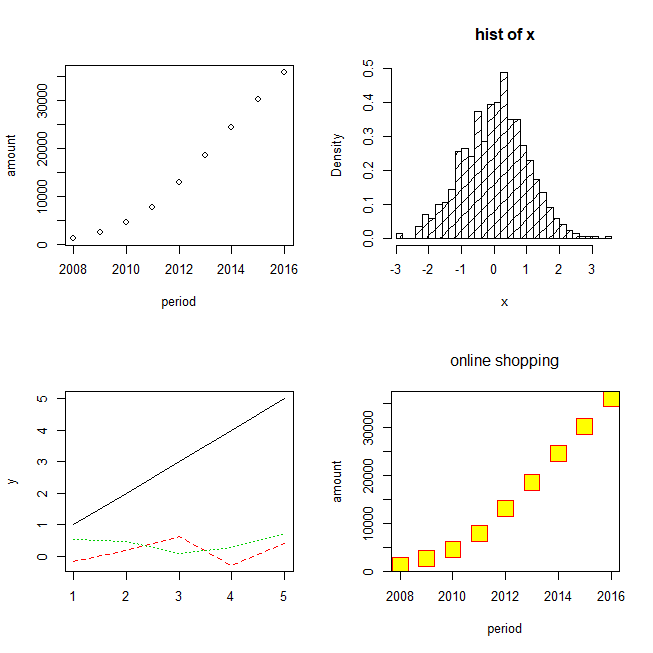展开全文par 函数 r语言
• 版权声明无须授权即可转载，请保留作者及以上版权声明可能是我理解能力有限，居然被par这个函数困扰了很久。今天就把我的学习心得分享给大家。咱们先来看几个效果图，先不讲虚的理论。1基本作图，先画一个dose--...

版权声明

无须授权即可转载，请保留作者及以上版权声明

可能是我理解能力有限，居然被par这个函数困扰了很久。今天就把我的学习心得分享给大家。

咱们先来看几个效果图，先不讲虚的理论。

1

基本作图，先画一个dose--drugA的关系图

dose

drugA

drugB

plot(dose, drugA, type="b")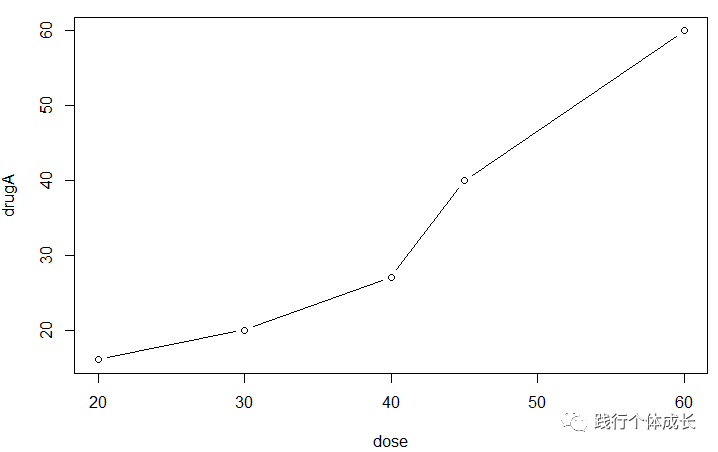我们发现，线型为实线，点为空心圆形。

2

接着修改参数,如：lty，pch。我们用par()函数修改这些参数。

opar

par(lty=2, pch=17)

plot(dose, drugA, type="b")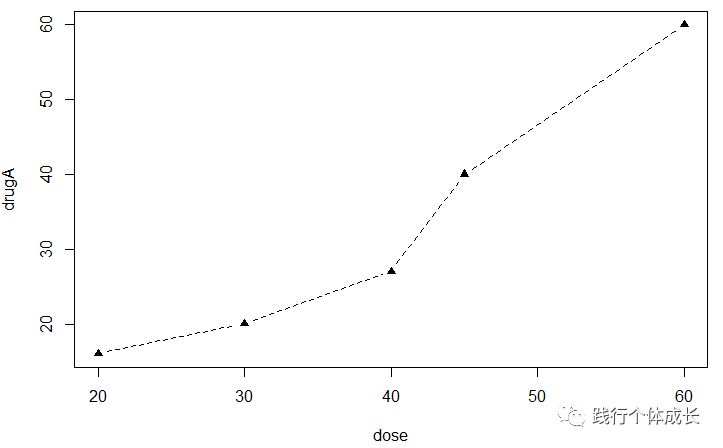我们发现用dose-drugA做的图，线型和符号都发生了变化。线型变为了虚线，图标变为实心黑色三角。

3

我们继续画dose--drugB的图

plot(dose, drugB, type="b")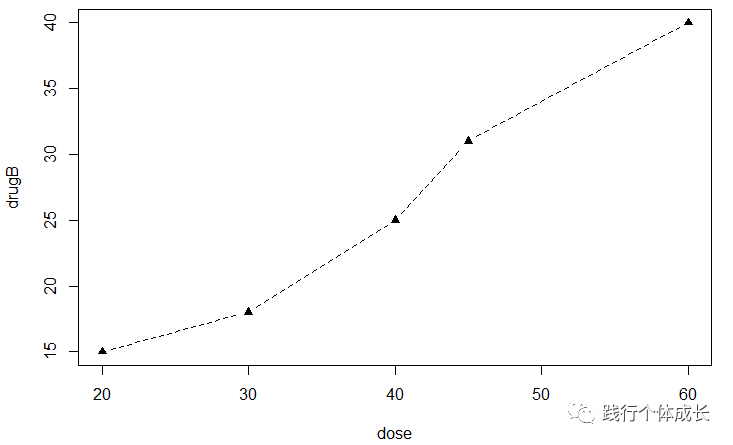我们发现dose--drugB做的图不是原来的实线，空心环形图，而是也变成了虚线，实心三角图。

这是因为par()函数修改了默认参数，在plot()函数里，你无须再写plot(lty=2, pch=17)这段，后面都会按这个默认参数执行plot绘图。那我们如果想回到最原始的默认参数，不想把lty与pch两个参数默认设为lty=2, pch=17怎么办呢？

4

这就要用到下面语句了

par(opar)

输入完上面语句，我们再来画一下dose--drugB的图看一下：

plot(dose, drugB, type="b")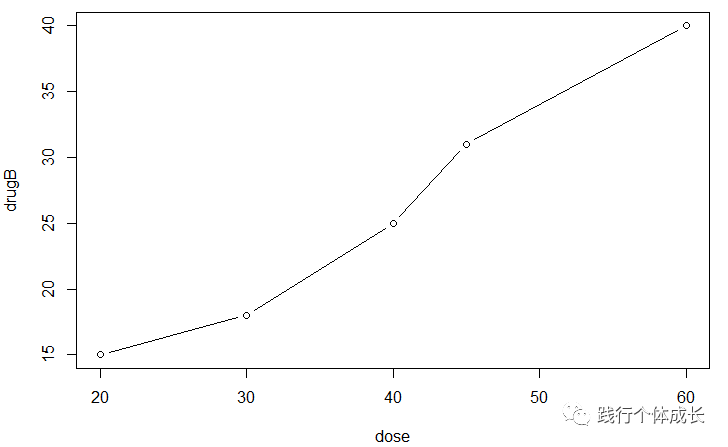图形又回到了默认的参数状态，实线空心圆的图。

注意：这里我们能恢复到原来的参数状态，是因为我们在执行par(lty=2, pch=17)语句前，先执行了opar

dose

drugA

drugB

plot(dose, drugA, type="b")

par(lty=2, pch=17)

plot(dose, drugA, type="b")

opar

par(opar)

plot(dose, drugB, type="b")

我们将得到下面这张图：也就是说opar

5

lty与pch这两个参数我们也可以直接用plot函数执行，但是这个只对当前作图有效。例如：

plot(dose, drugA, type="b"，lty = 2, pch = 17)用dose-drugA做的图，线型和符号也都发生了变化。

但如果我们不用par()函数，直接接着画dose--drugB的图

plot(dose, drugB, type="b")

我们发现，drugB还是空心圆直线图。

一些理论知识

下面讲一些别的关于par()的知识。很多时候R语言中默认的绘图参数并不能满足我们的需要，需要用par()函数进行提前设定，查看默认参数用par()

一、只能通过par()设置的参数

2， "fig", "fin",

3，"lheight",

4，"mai", "mar", "mex", "mfcol", "mfrow", "mfg",

5，"new",

6，"oma", "omd", "omi",

7，"pin", "plt", "ps", "pty",

8，"usr",

9，"xlog", "ylog",

10，"ylbias"

二、只能读取，不能进行设置。包括参数cin，cra，csi，cxy， din

三、剩下的参数除了函数par()外，还可以通过各种高级绘图函数进行设置，如函数plot，points，lines，abline，title，text，axis，image，box，contour，rect，arrows等，这些参数有："xlog" ,"ylog" , "adj"  , "ann"  , "ask"  ,"bg" , "bty" , "cex"  ,  "cex.axis" , "cex.lab"   "cex.main" , "cex.sub"  ,"cin"  ,"col" , "col.axis"  "col.lab"   "col.main"  "col.sub"  , "cra" , "crt"  ,  "csi"  , "cxy" ,"din" , "err" ,"family", "fg" ,"fig" , "fin" ,"font", "font.as", "font.lab" , "font.main" ,"font.sub" , "lab" ,"las" ,"lend" ,"lheight", "ljoin"  "lmitre"  "lty" , "lwd" ,  "mai" ,"mar" ,"mex","mfcol" ,"mfg" ,"mfrow","mgp" , "mkh", "new" ,  "oma" ,"omd" , "omi" , "page" , "pch","pin" ,"plt" , "ps","pty" ,"smo" ,"srt" ,"tck","tcl"    ,"usr" ,"xaxp","xaxs" ,"xaxt","xpd","yaxp","yaxs" ,"yaxt" ,"ylbias"

展开全文• R语言_par()函数用法

千次阅读 2021-05-22 02:54:28

前言:

微博参与话题 #给你四年时间你也学不会生信#

图形参数

1、符号和线条

pch：指定绘制点所使用的符号，取值范围[0, 24]，其中4是“差号”，20是“点”

cex：指定符号的大小。cex是一个数值，表示pch的倍数，默认是1.5倍

lty：指定线条类型。lty=1代表实线，2至6都是虚线，虚的程度不一样

lwd：指定线条宽度，默认值为lwd=1，可以适当修改1.5倍、2倍等

2、颜色

col：默认绘图颜色。某些函数(如lines、pie)可以接受一个含有颜色值的向量，并自动循环使用。

例如：col=c("red", "blue")需要绘制三条线，那么三条颜色分别为red、blue、red

col.axis：坐标轴刻度文字的颜色，不是坐标轴的颜色

col.lab：坐标轴标签(名称)的颜色

col.main：标题的颜色

col.sub：副标题的颜色

fg：图形的前景色

bg：图形的背景色

3、文本属性(用来指定字号、字体、字样)

cex.axis：坐标轴刻度文字的缩放倍数

cex.lab：坐标轴标签(名称)的缩放倍数

cex.main：标题的缩放倍数

cex.sub：副标题的缩放倍数

font：整数。用于指定字体样式。1常规、2粗体、3斜体、4粗斜体

4、图形尺寸与图形边界

pin：以英寸表示图形的宽和高

mai：以数值向量表示边界大小，顺序为"下、左、上、右"，单位为英寸

mar：以数值向量表示边界大小，顺序为"下、左、上、右"，单位为英分，默认值c(5, 4, 4, 2)+0.1

5、标题

可以使用函数title，格式为：

title(main = " ", sub = " ", xlab = " ", ylab = " ")

也可以直接把title里面的参数直接放在plot()里面

6、坐标轴

plot参数

axes=FALSE 将禁用全部坐标轴，框架和刻度全部没有了

xaxt="n" 禁用x轴的刻度线

yaxt="n" 禁用y轴的刻度线

xlim x坐标轴的范围，只写出最小值和最大值

ylim y坐标轴的范围，只写出最小值和最大值

例如：plot(1:10, xlim = c(-10, 10), ylim = c(0, 10)) xlim和ylim只是坐标轴的范围，与每个点的x和y坐标没有关系，也可以通过axis函数自定义axis(……)

side：一个整数。表示在图形的哪边绘制坐标轴(1=下，2=左，3=上，4=右)

at：一个数值向量，表示需要绘制刻度线的位置

labels：一个字符型向量(也可以是数值型)，表示刻度线旁边的文字标签(刻度值)，如果整个不写，则直接使用at的值

col：线条和刻度的颜色

lty：线条类型

las：标签的字体是否平行(=0)或者垂直(=2)坐标轴

tck：刻度线的长度(默认值-0.01，负值表示刻度在图形外，正值表示刻度在图形内侧)

7、参考线

abline(h=yvalues, v=xvalues)

例如：plot(1:10)

abline(h = c(1, 5)) #则在y=1和5处各有一条水平线

abline(v = c(1, 5)) #则在x=1和5处各有一条垂直线

8、图例(legend)

legend(location, title, legend, ……)

待续……

展开全文r语言中形成的c函数
• R语言————par函数

千次阅读 2019-12-16 23:00:00
【1】在一幅图开始之前先把作图参数保存到一个对象，比如op = par() 【2】在作这幅图的过程中用par()函数任意更改设置以适合需要 【3】作完图后，再用par(op)语句把之前保存的参数设置“释放”出来， 【4】这样...

为什么很多代码一开始先写一个par( ) ？

【这个函数会“永久性”改变作图设置，而我们有时并不想要这种功能，我们可能希望画下一幅图的时候，之前的参数可以被“还原”回来】

【1】在一幅图开始之前先把作图参数保存到一个对象中，比如op = par()

【2】在作这幅图的过程中用par()函数任意更改设置以适合需要

【3】作完图后，再用par(op)语句把之前保存的参数设置“释放”出来，

【4】这样，中间过程对图形参数的更改就不再会影响到下一幅图。

目前par()函数涉及到的图形参数大约有70个

或者这样子：

par(opar)用来还原默认变量环境。

展开全文• R语言笔记--par()函数详解

万次阅读 多人点赞 2017-01-17 11:37:56
R有着非常强大的绘图功能，我们可以利用简单的几行代码绘制出各种图形来，但是有时候默认的图形设置没法满足我们的需要，甚至会碰到各种各样的小问题：如坐标轴或者标题出界了，或者图例说明的大小或者位置遮挡住了...R par 图形
• 本课程旨在帮助学习者快速入门R语言： 课程系统详细地介绍了使用R语言进行数据处理的基本思路和方法。 课程能够帮助初学者快速入门数据处理。 课程通过大量的案例详细地介绍了如何使用R...r语言 编程语言 云计算/大数据 大数据
• 注：参考书籍《R作图–现代统计图形》谢益辉 par()函数的特点： par()函数可以用来设置或者获取图形参数。 函数par()可以全局设置图形参数，而在具体作图函数plot() 或 lines()是临时设置图形参数。二者的区别是：...r语言
• R语言 公共参数列表par()手册

千次阅读 2018-08-15 16:24:41
初用R，发现在用基础包绘图时不同的画图函数很多参数都通用的，每次遇见都要去R中查help文件，所以在此借鉴论坛优秀的par解说，整理成手册，加深自己记忆的同时，方便以后查找参数~ par参数涉及颜色、字体、...
• R语言函数（1）-par()函数

千次阅读 2020-05-31 16:20:48
R语言中可以有多个函数来实现此要求。这里先介绍一下绘图参数par函数的使用。 Rpar()函数可以将绘图区分割成规则的几个部分。 多图环境用参数mfrow或参数mfcol来设定，如： par(mforw=c(3,2)) 则是在同一绘图...数据分析
• R语言（绘图入门）

千次阅读 2020-12-22 22:19:44r语言mfrow全程
• R语言par函数设置图像个数、边界、颜色等实战 ...使用par函数设置或查询R语言中的图形参数。 #par函数设置多图可视化 par(mfrow = c(2, 3)) # Multiple plots plot(1:10) # 1st plot plor语言 数据挖掘 机器学习 自然语言处理 人工智能
• #预先在数据框分块,2*2分块 par(mfrow=c(2,2),mar=c(3,3,2,1)) par(del) #画好需要关闭 legend(x,y,fill=v,col=v,lty=v, lwd=v,pch=v) 在当前图的特定位置增加图例（legend）。 #legend( , fill=v)填充盒子的...
• R中使用函数par()，layout()可以很容易组合多副图为一副总括图形。 一、par() 用法 在par 函数使用图形参数mfrow=c(nrows,ncols)来按照行填充的行数为nrows，列数为ncols的图形矩阵。也可以使用mfcol=c(nrows,...R语言 par layout fig
• R语言par(fig=)函数的详细解释

万次阅读 热门讨论 2017-12-08 11:14:37
图形布局的精细控制 可能有很多时候，你想通过排布或叠加若干图形来创建单幅的、有意义的图形，这需要有对 ...opar<-par(no.readonly = TRUE) par(fig=c(0,0.8,0,0.8)) plot(mtcars$wt,mtcars$mpg,xlab =
• 不同于plot()函数，par()函数的参数除个别外一旦设定，除非再次更改或重启R，否则就会对后续所有的绘图命令起作用。在设置参数之前，可以使用以下代码保存绘图系统的默认参数： # 储存当前参数 opar <- par(no....r语言
• R语言画图——par()参数介绍

千次阅读 2015-06-12 11:03:54
这两天一直在学R的画图，从最基础的学起，争取弄懂常见常用参数的用法。...R中设置图形参数--函数par()详解 http://blog.sina.com.cn/s/blog_6caea8bf0100yk4h.html R绘图基础（一）  http://blog.163.com/R plot par
• par(mfcol = c( 3 , 3 ) ) par(mgp = c( 3 , 1 , 1 ) ) plot( 1 : 10 , ylab= "mgp = c(3, 1, 1)" , xlab= "mgp = c(3, 1, 1)" , cex.lab= 1.5 ) par(mgp = c( 3 , 2 , 0 ) ) plot( 1 : 10 , ylab= ...r语言 par
• R 语言图形函数par()参数应用举例函数par()的参数可以分为三大类：(1)只可以查询而不可以修改的参数, 即 readonly 参数："cin", "cra", "csi", "cxy", "din"和 "page"。(2 )只能通过 par 函数进行设置的参数: "ask...fig r函数
• R有着非常强大的绘图功能，我们可以利用简单的几行代码绘制出各种图形来，但是有时候默认的图形设置没法满足我们的需要，甚至会碰到各种各样的小问题：如坐标轴或者标题出界了，或者图例说明的大小或者位置遮挡住了...r语言 编程语言 数据分析
• R语言par()函数

千次阅读 2014-06-29 19:09:00
• 备注：学习备忘1、使用par(mfrow=c(2,2))即可>par(mfrow=c(3,3))> plot(Nile)> plot(Nile)> plot(Nile)> plot(Nile)> plot(Nile)> plot(Nile)> plot(Nile)> plot(Nile)> plot(Nile) ...
• R 绘图参数设置函数par()详解

千次阅读 2015-05-23 10:32:52
par()函数是R里有关绘图的重要函数之一，以下是对par()函数说明内容的一些整理。 描述： par函数用于设定或询问绘图参数。参数设定可通过par(参数名 = 取值)或par(赋值参数列表)的形式进行。 用法： par(..., ...r语言
• 二者返回的函数区别为：colorRamp()返回的函数像grey()一样，入参为[0,1]之间的数列，数列数字个数即为函数返回的颜色板色彩数。colorRampPalette()返回的参数则像rainbow()一样，入参为希望返回颜色板色彩的数量...r语言mfrow全程
• 我们在使用R语言制作可视化图形时，往往遇到一些标签、颜色上修改的小问题，今天博主就自己的一些经验，给大家分享一下。 如图，这是博主在根据企业财报数据做分析时用到的部分数据。如果我们想从不同技术板块来看看...r语言 开发语言 数据分析
• 10 * sin(r)/r } z (x, y, f) z[is.na(z)] <- 1 op <- par(bg = "white") persp(x, y, z, theta = 30, phi = 30, expand = 0.5, col = "lightblue") persp(x, y, z, theta = 30, phi = 30, expand = 0.5, col = ...r语言 算法
• 方法一 library(ggplot2) library(patchwork) p1 <- ggplot(iris,aes(x=Sepal.Length))+geom_histogram(fill='#58a6a6') p2 <- ggplot(iris,aes(x=Sepal.Width))+geom_histogram(fill='#1c595a') ...r语言 ggplot2 par
• a[c(3:5)，2] 矩阵编辑：#矩阵合并（a1(a,c(11,12))） (a2(a,c(11:15))) #删除矩阵元素 a5[-1,] #删除矩阵的第一行 矩阵的运算：colSums()-对矩阵的各列求和 rowSums()-对矩阵的各行求和 colMeans()-对矩阵...r语言中mpg数据...